Processing ......FreeComputerBooks.com Links to Free Computer, Mathematics, Technical Books all over the World

Bayesian Data Analysis
Top Free C Programming Books 🌠 - 100% Free or Open Source!
• Title: Bayesian Data Analysis
• Author(s) Andrew Gelman, John Carlin, Hal Stern, David Dunson, Aki Vehtari, and Donald Rubin
• Publisher: Chapman and Hall/CRC; 3rd edition (2013); eBook (Errors Fixed Edition, 2021)
• Hardcover: 675 pages
• eBook: PDF (677 pages)
• Language: English
• ISBN-10/ASIN: 1439840954
• ISBN-13: 978-1439840955Book Description

This classic book is widely considered the leading text on Bayesian methods, lauded for its accessible, practical approach to analyzing data and solving research problems. Bayesian Data Analysis, Third Edition continues to take an applied approach to analysis using up-to-date Bayesian methods.

The authors - all leaders in the statistics community - introduce basic concepts from a data-analytic perspective before presenting advanced methods. Throughout the text, numerous worked examples drawn from real applications and research emphasize the use of Bayesian inference in practice.

New to the Third Edition:

• Four new chapters on nonparametric modeling
• Coverage of weakly informative priors and boundary-avoiding priors
• Updated discussion of cross-validation and predictive information criteria
• Improved convergence monitoring and effective sample size calculations for iterative simulation
• Presentations of Hamiltonian Monte Carlo, variational Bayes, and expectation propagation
• New and revised software code
• N/A
Reviews, Ratings, and Recommendations: Related Book Categories: Read and Download Links:Similar Books:
•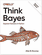O'Reilly® Think Bayes: Bayesian Statistics in Python

If you know how to program, you're ready to tackle Bayesian statistics. With this book, you'll learn how to solve statistical problems with Python code instead of mathematical formulas, using discrete probability distributions rather than continuous mathematics.

•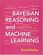Bayesian Reasoning and Machine Learning (David Barber)

This practical introduction is ideally suited to computer scientists without a background in calculus and linear algebra. You'll develop analytical and problem-solving skills that equip them for the real world. Numerous examples and exercises are provided.

•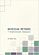Bayesian Methods for Statistical Analysis (Borek Puza)

Bayesian methods for statistical analysis is a book on statistical methods for analysing a wide variety of data. It contains many exercises, all with worked solutions, including complete computer code.

•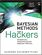Bayesian Methods for Hackers: Probabilistic Programming

This book illuminates Bayesian inference through probabilistic programming with the powerful PyMC language and the closely related Python tools NumPy, SciPy, Matplotlib, through practical examples and computation - no advanced mathematics required.

•An Introduction to Bayesian Thinking (Merlise Clyde, et al.)

This book provides an introduction to Bayesian inference in decision making without requiring calculus. It may be used on its own as an open-access introduction to Bayesian inference using R for anyone interested in learning about Bayesian statistics.

•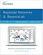Bayesian Networks and BayesiaLab (Stefan Conrady, et al.)

This practical introduction is geared towards scientists who wish to employ Bayesian Networks for applied research using the BayesiaLab software platform. It can serve as a self-study guide for learners and as a reference manual for advanced practitioners.

Book Categories
 :All CategoriesTop Free BooksRecent BooksMiscellaneous BooksComputer EngineeringComputer LanguagesComputer ScienceData Science/DatabasesJava and Java EE (J2EE)Linux and UnixMathematicsMicrosoft and .NETMobile ComputingNetworking and CommunicationsSoftware EngineeringSpecial TopicsWeb Programming
Other Categories